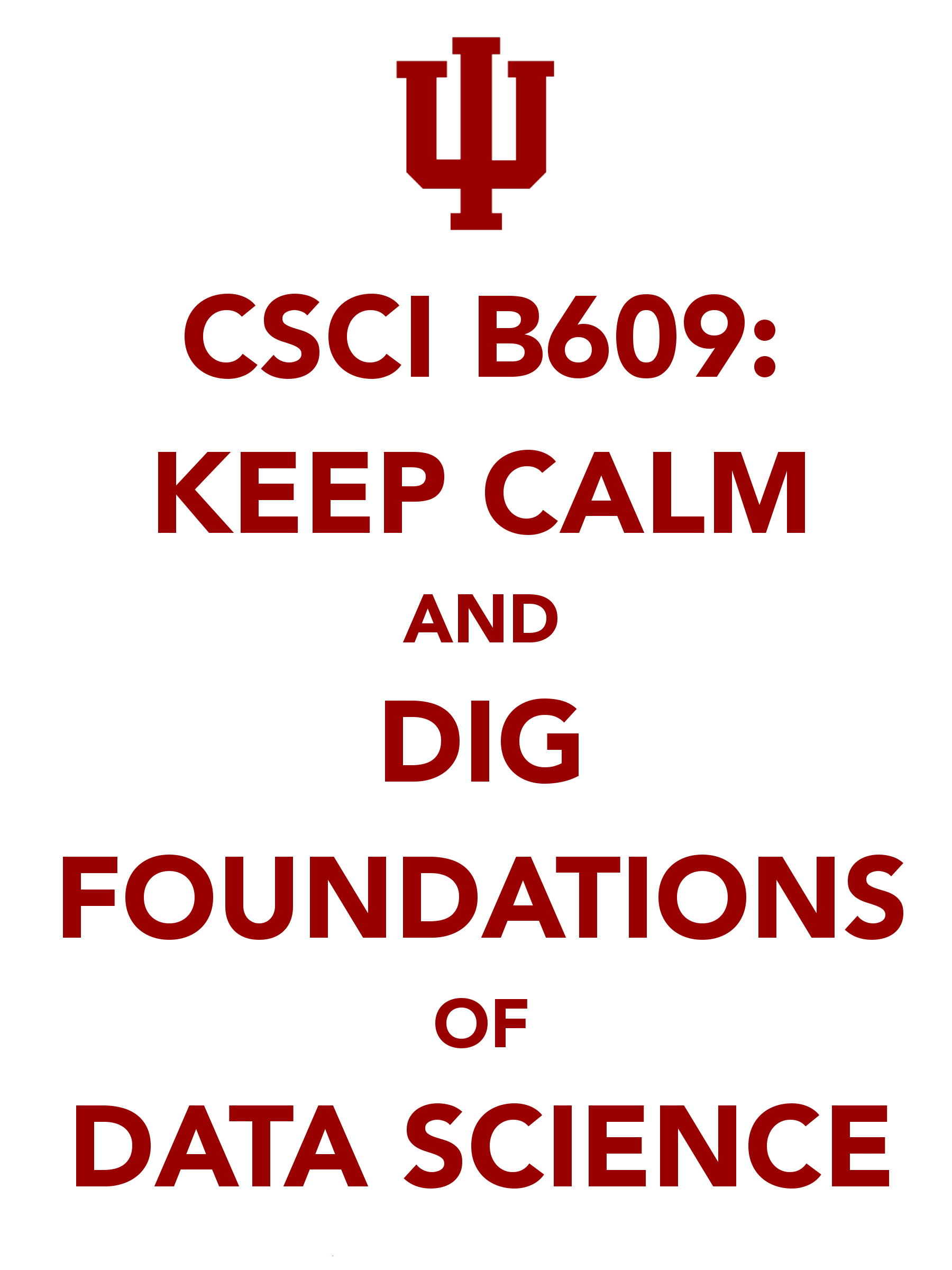# CSCI-B609: Foundations of Data ScienceWhat? This class will give you a mathematical toolkit that can be applied to problems in data anslysis. Target audience are students interested in algorithms, statistics, machine learning, data mining and related areas. Who? Grigory Yaroslavtsev. When? Fall 2016, MW 16:00 – 17:15. Where? Ballantine Hall, 310. Need permission? Please, send me an e-mail with relevant coursework you have taken. In most cases permission will be granted. Grading? The class will be graded based on home assignments and a final project. TA? Md. Lisul Islam, IU id: islammdl. Office hours? Held by the Md. Lisul Islam on Tuesdays, 12:30–2pm at Lindley Hall, 125. Prerequisites? There are no formal prerequisites, but you should be familiar with the basics of algorithm design and analysis, discrete mathematics, probability and have a strong mathematical background. Textbook? This class is primarily based on the emponimous textbook by Blum, Hopcroft and Kannan which is available here. More questions? In addition to Canvas we are using Piazza for questons. Sign up: here. Please, don't use my personal e-mail for questions.

# Abstract

This class covers a rapidly evolving area. Related classes at other universities:

# Lectures

• Week 1. [Slides (pptx, pdf)] Introduction. Probablity basics. Probabilistic inequalities and concentration bounds.
• Week 2. [Slides (pptx, pdf)] Properties of high-dimensional space. Surface area and volume of the unit ball in high dimensions, distribution of volume. Near-orthogonality of random high-dimensional vectors. Sampling from a unit ball. Gaussian concentration.
• Week 3. [Slides (pptx, pdf)] Nearest neighbor search and random projections. Separating high-dimensional Gaussians. Fitting a high-dimensional Gaussian (maximum likelihood).
• Week 4. [Slides (pptx, pdf)] Singular Value Decomposition and Best-Fit Subspaces. Greedy construction of the SVD. Low-rank approximation (Frobenius and spectral norm). Power method.
• Week 5. [Slides (pptx, pdf)] Faster power method. Applications of SVD (centering data for SVD and PCA). Separating mixtures of Gaussians. HITS and document ranking.
• Week 6. [Slides (pptx, pdf)] Random walks and Markov chains. Stationary distribution. Fundamental theorem of Markov chains. Introduction to machine learning: distributional batch setting and uniform convergence. Online learning and perceptron algorithm.
• Week 7. [Slides (pptx, pdf)] VC-dimension and Sauer's lemma. VC-dimension of various classes and proof of the VC-theorem.
• Week 8. [Slides (pptx, pdf)] Constrained convex optimization. Gradient descent. Online and stochastic gradient descent. VC-dimension of combinations of classes. Boosting.
• Week 9. [Slides (pptx, pdf)] Introduction to streaming. Morris' algorithm. Approximate median. AMS-sampling. Frequency moments. Distinct elements. Count-Min sketch.
• Week 10. [Slides (pptx, pdf)] Graph sketching: connectivity, k-connectivity, bipartiteness, cut sparsification.
• Week 11. [Slides (pptx, pdf)] L0-sampling, L1-sparse recovery. CountSketch.
• Week 12. [Slides (pptx, pdf)] Massively parallel algorithms: sorting, graph connectivity, algorithms for geometric data.
• Week 13. [Slides (pptx, pdf)] Lp-testing.

# Homework

• Homework 1. (due September 25, 11:59 pm EST) [Source (tex), Compiled (pdf)]
• Homework 2. (due October 17, 11:59 pm EST) [Source (tex), Compiled (pdf)]
• Homework 3. (due November 21, 11:59 pm EST) [Source (tex), Compiled (pdf)]
• Homework 4. (due December 05, 11:59 pm EST) [Source (tex), Compiled (pdf)]

# Project

Details about the project: [Slides (pptx, pdf)]. Key dates:
• 1-page project proposal due on October 31, 11:59 EST.
• Full project submission due on December 09, 11:59 EST.